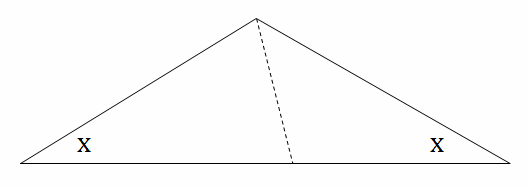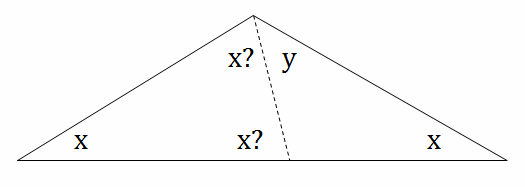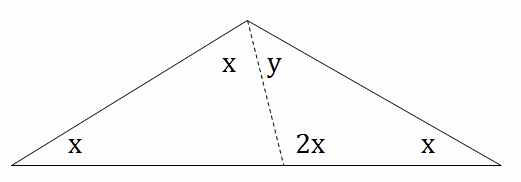### 數學題: 三角形的角度1. x = y

$$x = 45$$

$$x + y = 2x = 90 \rightarrow$$ 是等腰直角三角形。

2. 2x = y

$$x = 36$$

$$x + y = x + 2x = 3x = 108 \rightarrow$$ 鈍角是 108 度。